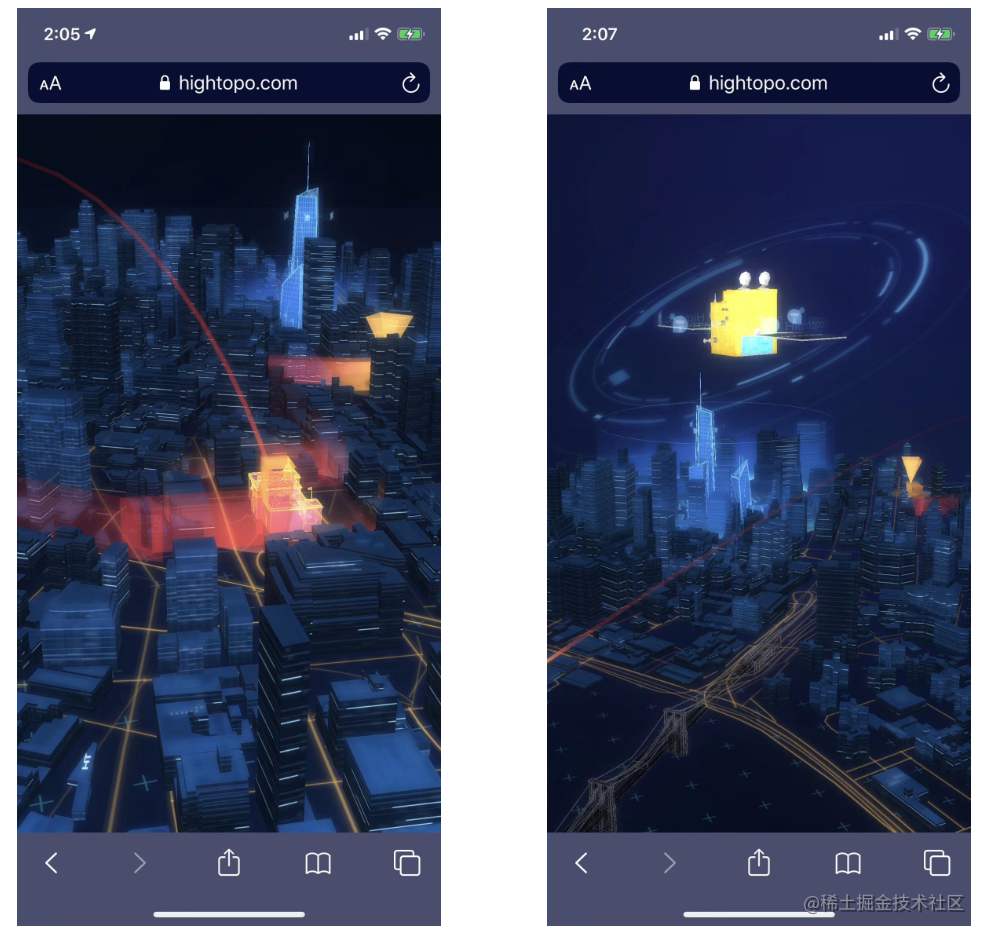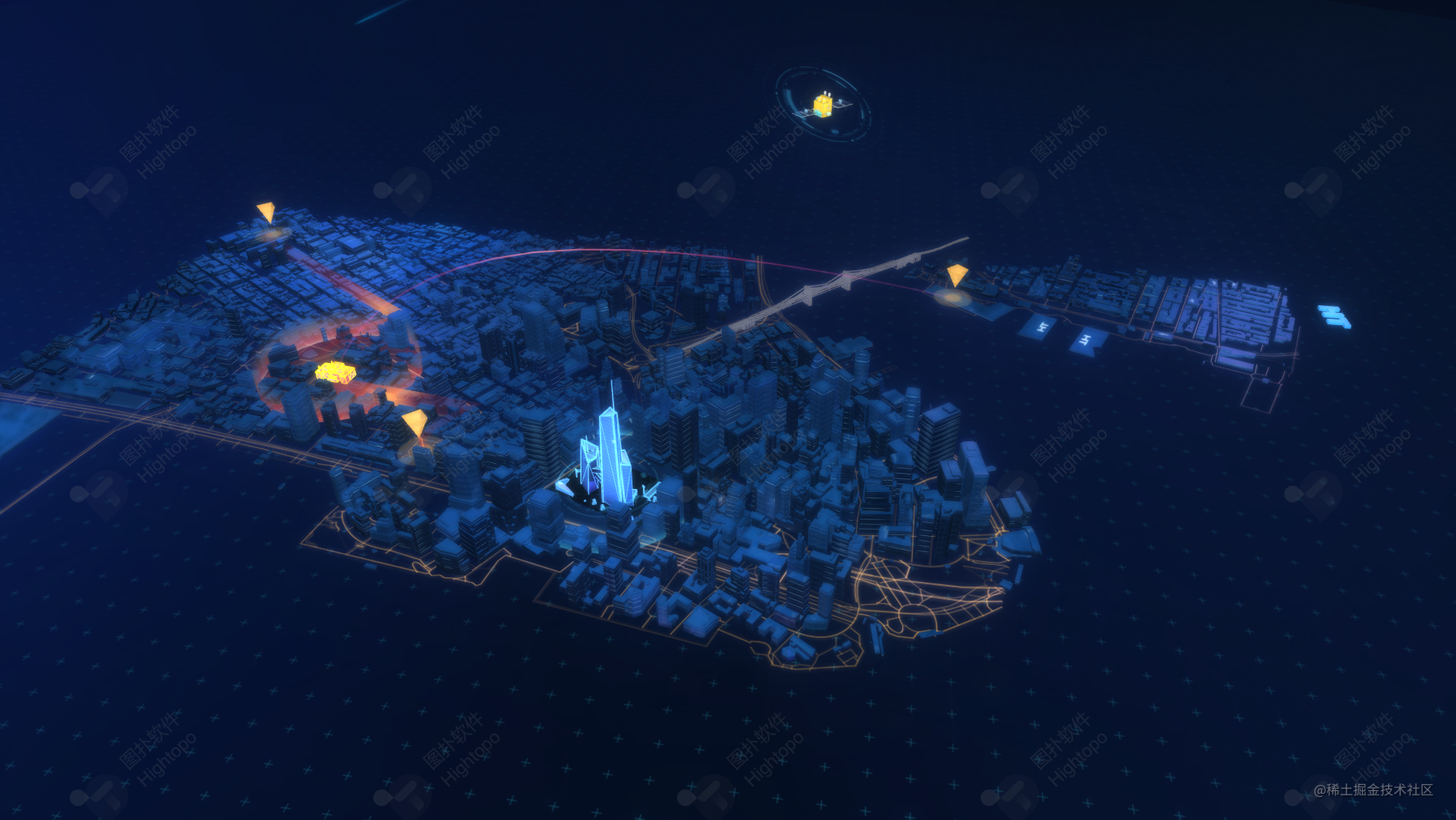# 基于 HTML5 WebGL 构建智能城市 3D 场景

## 前言

demo 地址：智慧城市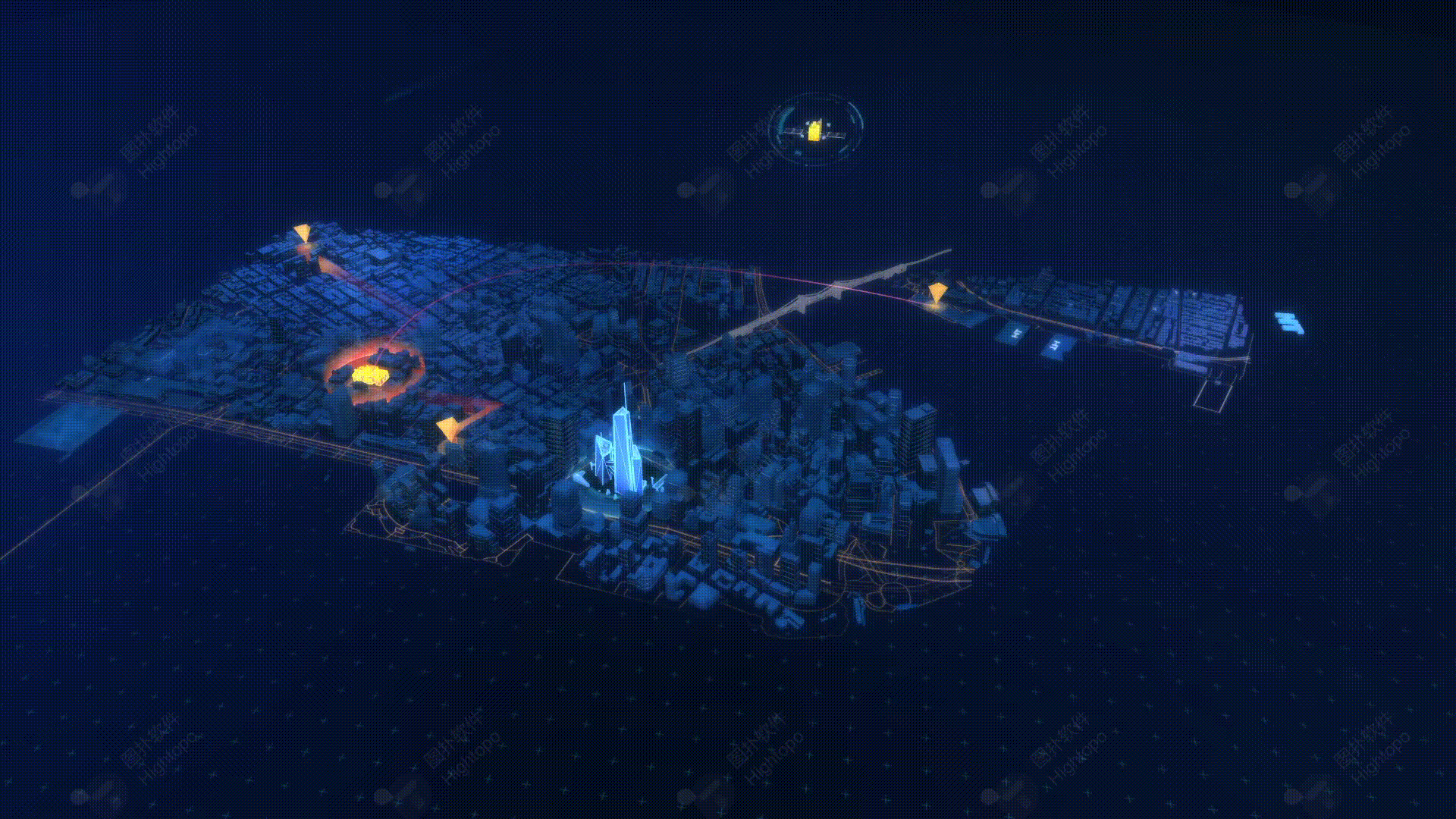## 代码实现

``````const g3d = new ht.graph3d.Graph3dView();
const dm3d = g3d.dm();

``````g3d.deserialize('scenes/ny.json', () => {
g3d.setEye([1132.8386351821287, 1916.836416970022, 1479.5345608290288]);
g3d.setCenter([519.9741236104874, 273.4741921410557, -319.58669041297884]);
g3d.setSkyBox(dm3d.getDataByTag('skyBox'));

// 获取扩散效果的图元
scaleList.push(
dm3d.getDataByTag('scaleBlue'),
dm3d.getDataByTag('scaleRed')
);
···

// 开始动画
startAnim();
});复制代码``````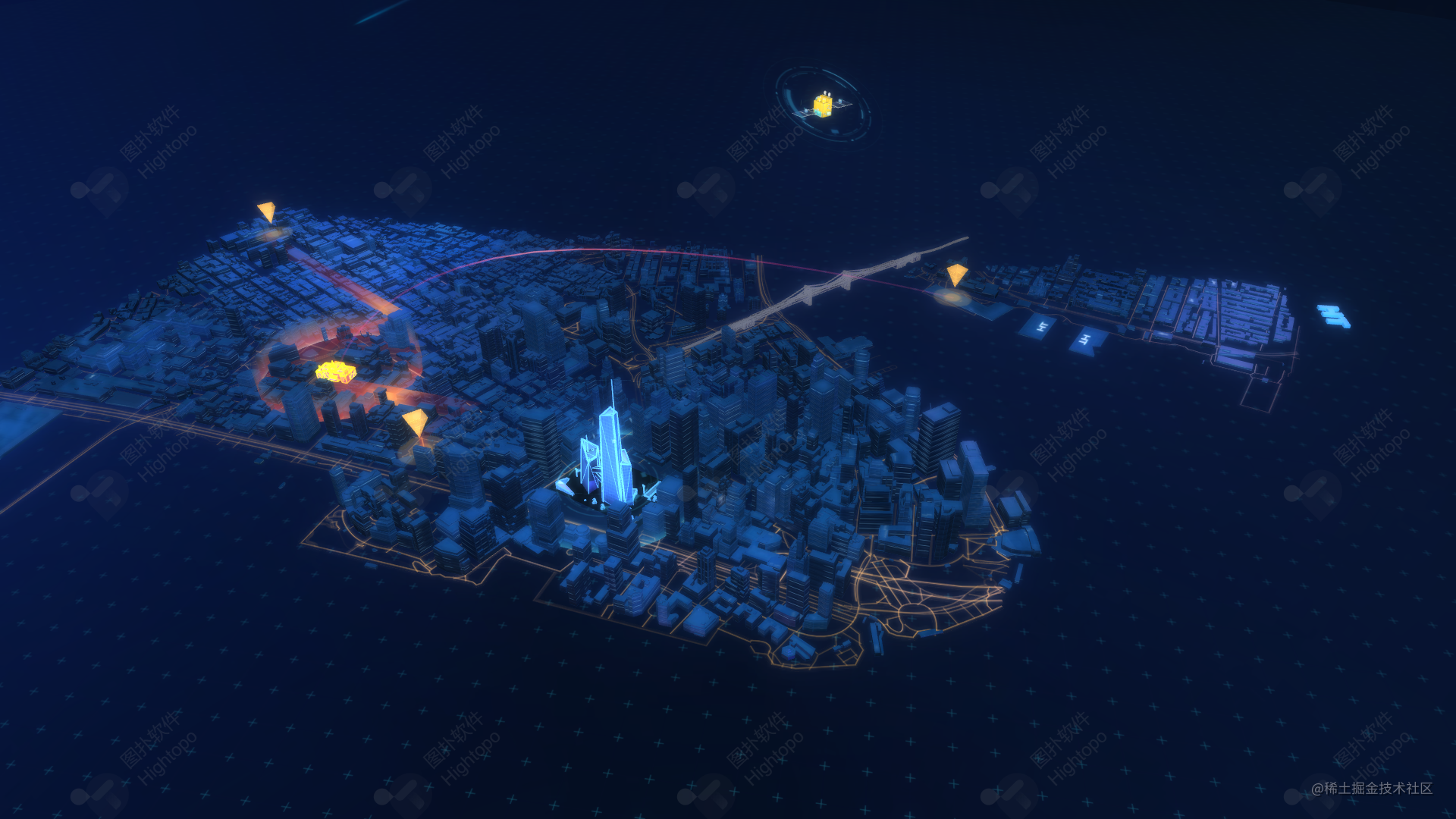``````ht.Default.startAnim({
// 动画帧数
frames: 12,
// 动画帧间隔毫秒数
interval: 10,
// 动画缓动函数，默认采用 ht.Default.animEasing
easing: function(t){ return t * t; },
// 动画结束后调用的函数
finishFunc: function(){ console.log('Done!') },
// action 函数必须提供，实现动画过程中的属性变化
action: function(v, t){
// 此例子展示将节点 node 从位置 p1 动画到位置 p2
node.setPosition(
p1.x + (p2.x - p1.x) * v,
p1.y + (p2.y - p1.y) * v
);
}
});复制代码``````

ht的动画手册可以参照：动画手册

1. 波纹效果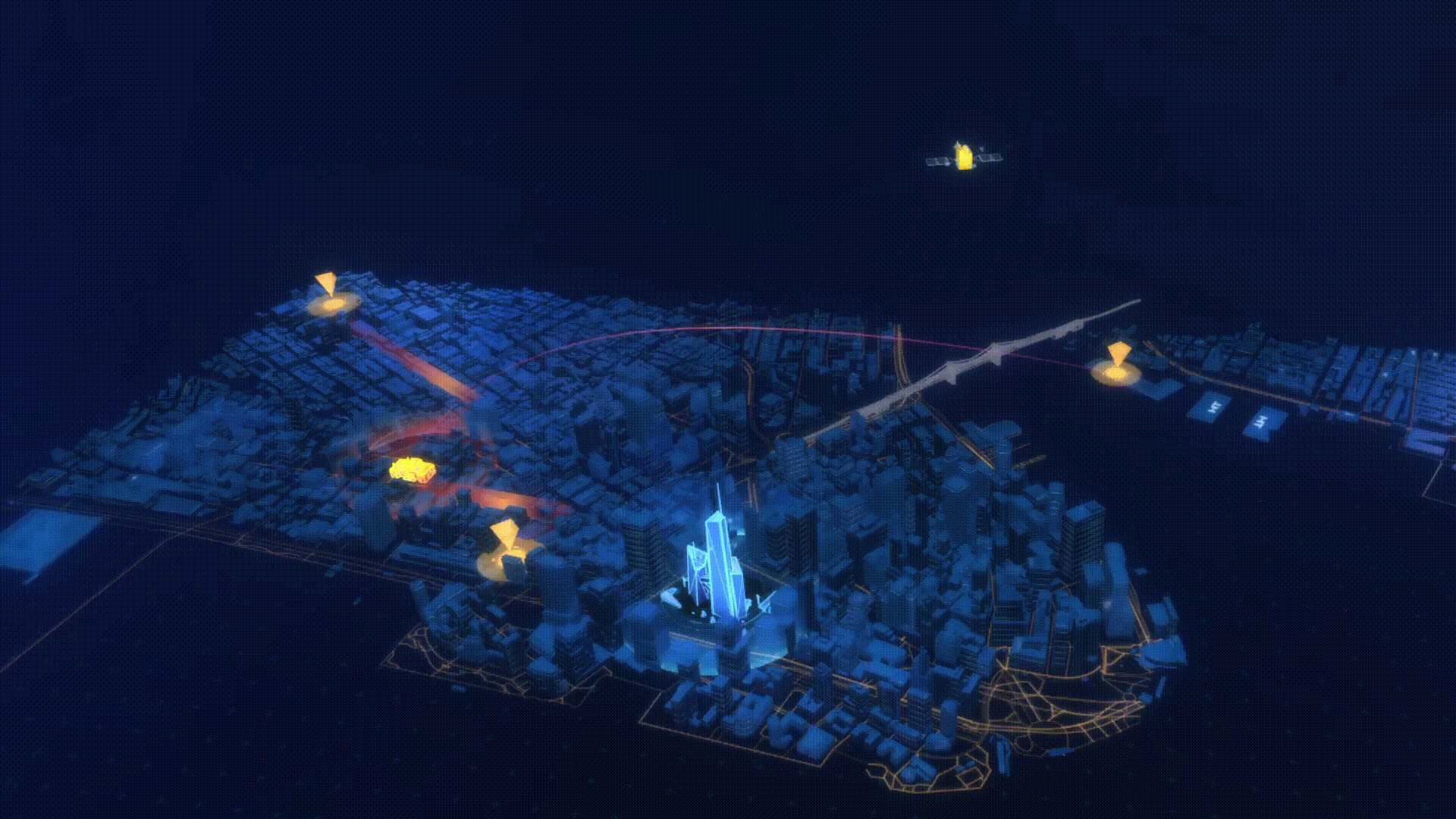``````function waveScale(obj, dlt, max, min) {
obj.forEach(node => {
// 扩散半径增加
let s = node.getScaleX() + dlt;
// 扩散半径大于最大值的时候, 重置为最小值
if (s >= max) s = min;
// 设置 x,y,z 方向的缩放值
node.setScale3d(s, s, s);
});
}
function startAnim() {
ht.Default.startAnim({
frames: Infinity,
interval: 20,
action: () => {
// 扩散蓝和扩散红
waveScale(scaleList, dltScale, maxScale, minScale);
}
});
}复制代码``````

2. 旋转效果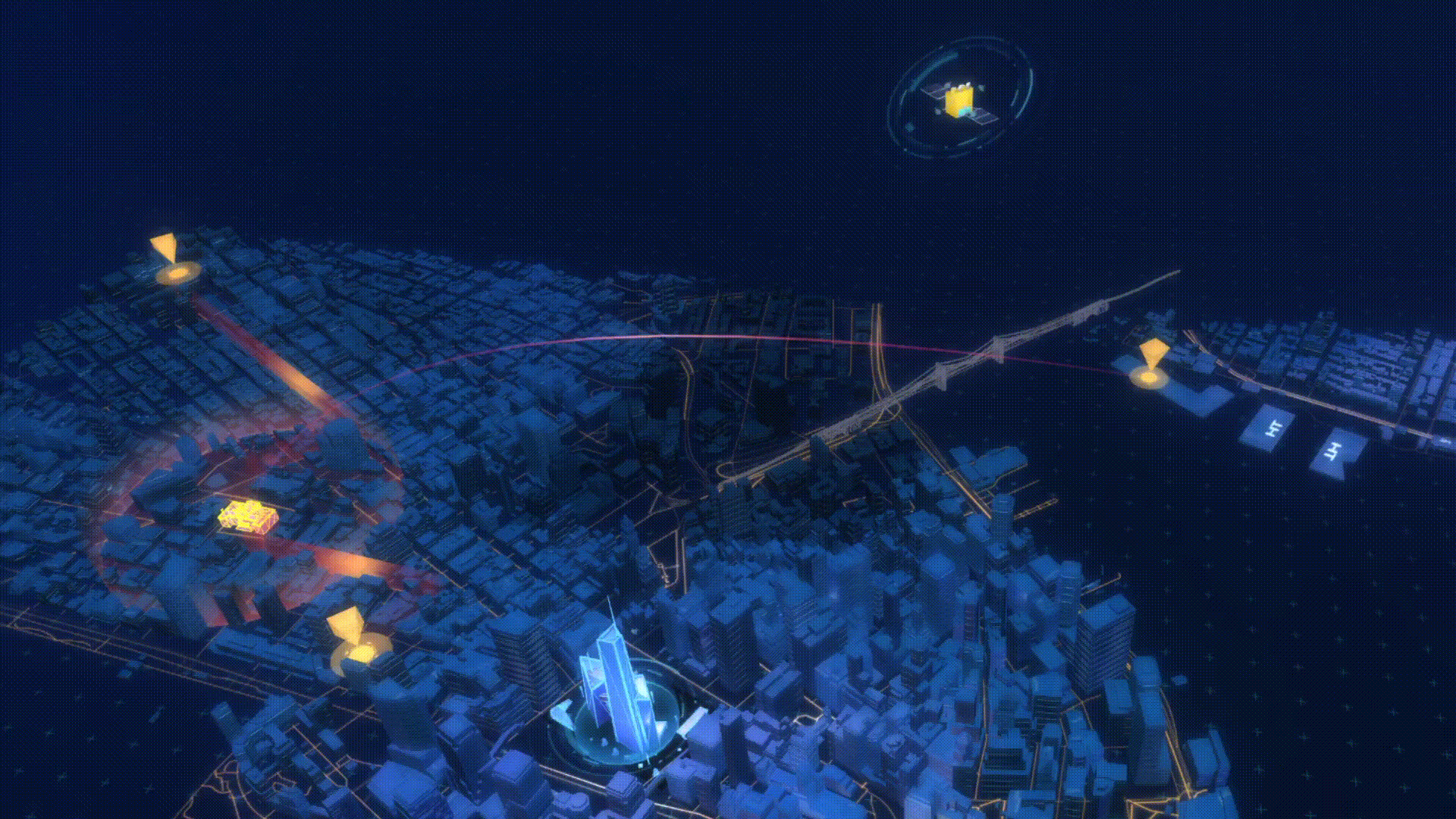``````function rotateAction(obj, dlt) {
// 获取图元旋转弧度值，没有就置零
let rotation = obj.getRotation() || 0;
// 每帧弧度增加
obj.setRotation(rotation + dlt);
}
function startAnim() {
ht.Default.startAnim({
frames: Infinity,
interval: 20,
action: () => {
// 卫星转动
rotateAction(star, dltRoattion);
}
});
}复制代码``````

3. 流动效果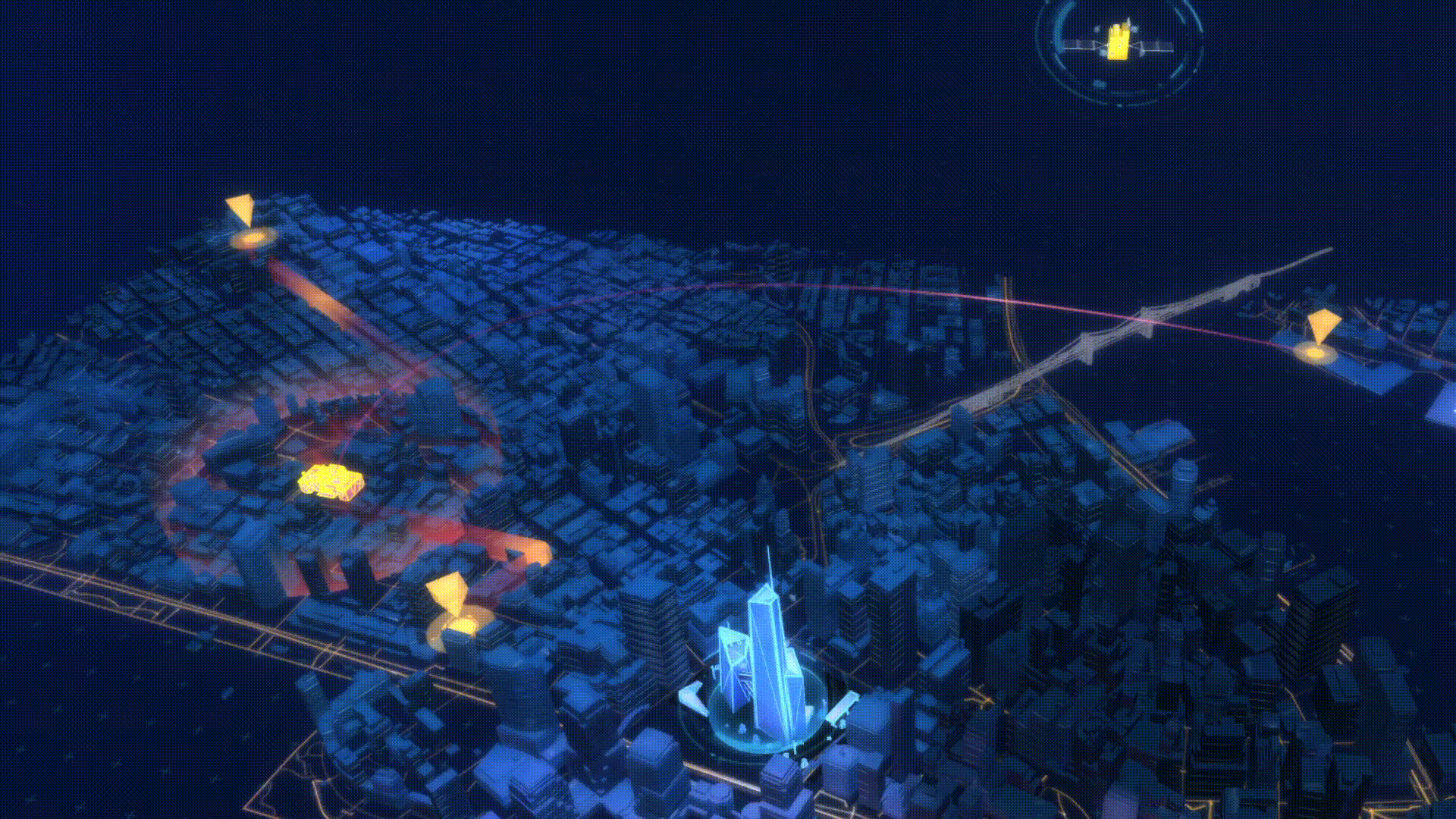``````function uvFlow(obj, dlt) {
// 改变贴图 uv 坐标实现流动效果
let offset = obj.s('all.uv.offset') || [0, 0];
obj.s('all.uv.offset', [offset + dlt, offset]);
}
function startAnim() {
ht.Default.startAnim({
frames: Infinity,
interval: 20,
action: () => {
// 小路流动效果
}
});
}复制代码``````

4. 浮动效果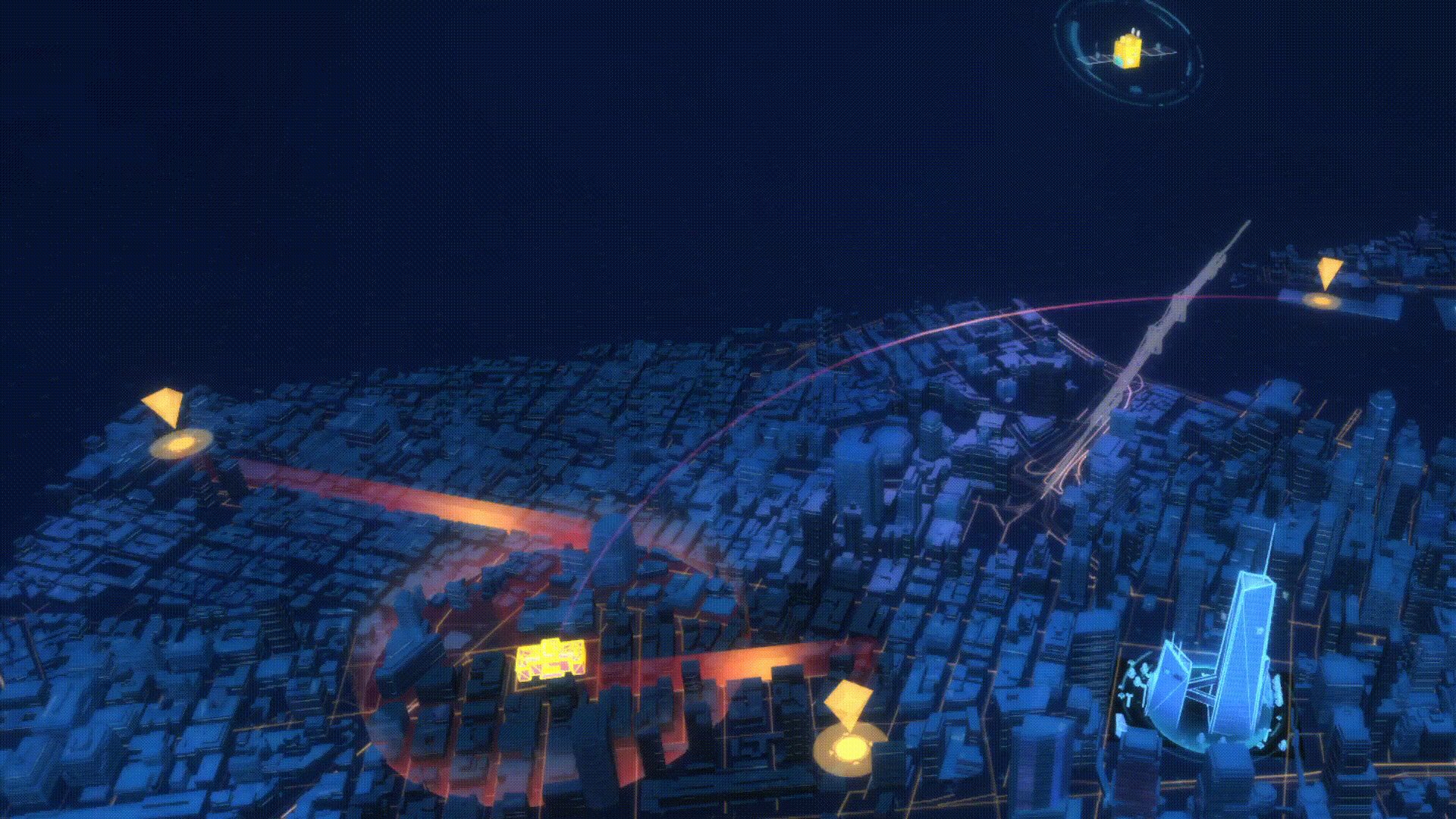``````function blockFloat(obj, dis, dlt) {
obj.forEach(node => {
let startE = node.a('startE');
if (startE == null)
// 获得图元在 y（高度）方向上的值
node.a('startE', startE = node.getElevation());
let float = node.a('float') || 0;
// 设置 status 为方向
let status = node.a('status') || 1;
node.setElevation(startE + dis * float);
if (float > 1 || float < 0)
// 超出阀值则改变方向
node.a('status', status = -status);
float += dlt * status;
// 重新设置图元高度
node.a('float', float);
});
}
function startAnim() {
ht.Default.startAnim({
frames: Infinity,
interval: 20,
action: () => {
// 消防标志浮动效果